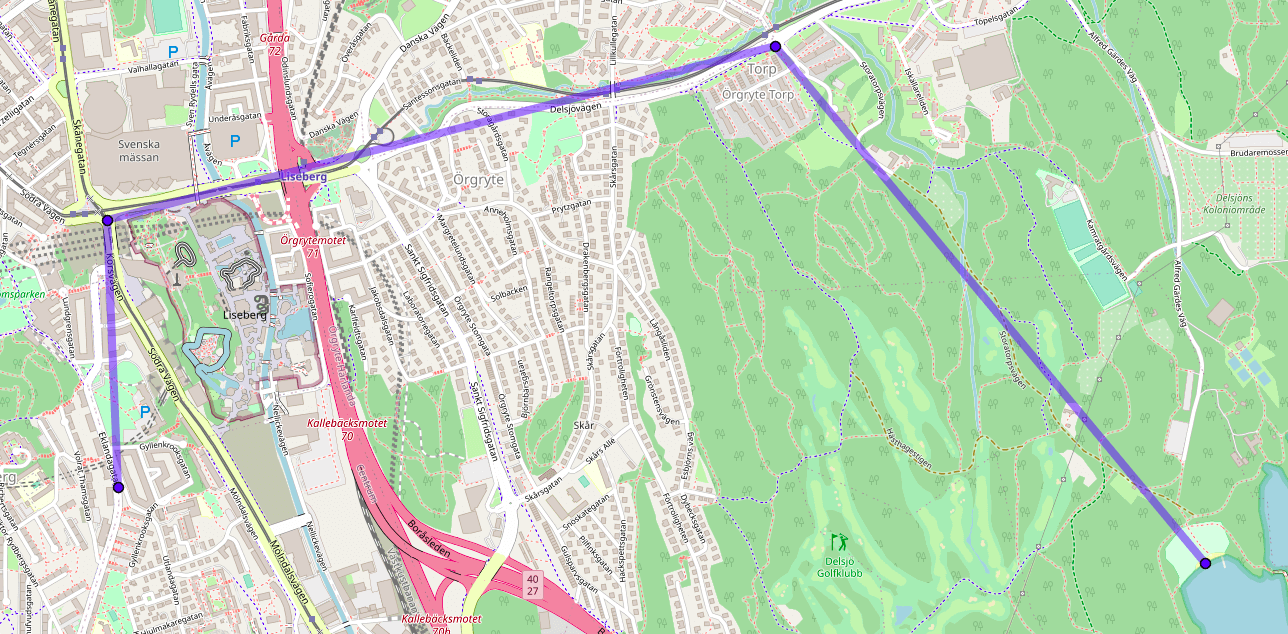# Sampling points along a lat,lon path

Here’s a path from Chalmers University to the beach at Stora Delsjön:The path consists of three segments, which can be defined by 4 points.

The problem of sampling along a path is to calculate `n` points that all lie on the path and are equally spaced along the path from each other. Some applications for this include

• Interpolating an irregularly-sampled path (like a GPX file of a run) into a regularly-sampled approximation (like for an elevation profile of the run).
• Line-of-sight calculation: what’s the highest elevation between two points?

With problems like this it’s important to avoid working with lat/lon angular coordinates directly, as the earth isn’t a perfect circle. And in general, I like to use libraries as they will handle all sorts of easy mistakes (like wrapping longitudes around the dateline). In python, the geographiclib has all the functions needed to handle paths and points on geoids, albeit with a non-pythonic api.

I’ll start by defining the path, and let’s calculate 8 intermediary points (which will include the ends).

``````import numpy as np
import mplleaflet
from geographiclib.geodesic import Geodesic

path = [
(57.6905, 11.9882),
(57.6966, 11.9877),
(57.7006, 12.0164),
(57.6888, 12.0348),
]
n_samples = 8
``````

WGS84 is an approximation of the actual shape of the earth. I’ll calculate distance as if travelling over this shape.

``````# Distance between each path coord.
geod = Geodesic.WGS84
path_distance = 
for (start_lat, start_lon), (end_lat, end_lon) in zip(path[:-1], path[1:]):
path_distance.append(geod.Inverse(start_lat, start_lon, end_lat, end_lon)['s12'])
``````

The distance of each sampled point along the line will be between 0 and the total distance between all path coordinates.

``````path_distance_cum = np.cumsum(path_distance)
point_distance = np.linspace(0, path_distance_cum[-1], n_samples)
``````

Now we need to first find which path segment each point lies on, then offset the point from the start of the segment by the required distance.

``````points = []
for pd in point_distance:

# Find segment with.
i_start = np.argwhere(pd >= path_distance_cum)[:, 0][-1]

# Early exit for ends.
if np.isclose(pd, path_distance_cum[i_start]):
points.append(path[i_start])
continue
elif np.isclose(pd, path_distance_cum[-1]):
points.append(path[-1])
continue

# Distance along segment.
start_lat, start_lon = path[i_start]
end_lat, end_lon = path[i_start + 1]
pd_between = pd - path_distance_cum[i_start]

# Location along segment.
line = geod.InverseLine(start_lat, start_lon, end_lat, end_lon)
g_point = line.Position(pd_between, Geodesic.STANDARD | Geodesic.LONG_UNROLL)
points.append((g_point['lat2'], g_point['lon2']))
``````

And the result: